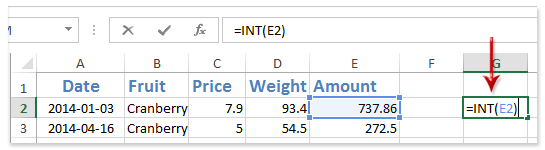I have an excel formula that is producing a lot of decimal places and I cannot reduce them using the format cell -> numbers -> decimal places options. Here is the formula.

Cell named V01_MIN V01_MIN =MIN(6:6) Has a value of 2

Cell named V01_MAX V01_MAX =MAX(6:6) Has a value of 1800

Cell named V01_A V01_A =1-V01_MIN*V01_B Has a value of 0.889877642

Cell named V01_B V01_B =99/(V01_MAX-V01_MIN) Has a value of 0.055061179

```X6=723
X7=V01_A+V01_B*X6 (value of 40.69911012)
X8=1
X9=X7*X8 (value of 40.69911012)
X10=1
X11=X9*X10 (value of 40.69911012)
X13==CONCATENATE(X12,", ",X11)
```

The final results of X13 are:

```V01, 1162, 40.6991101223582
```

I want them to be:

```V01, 1162, 40.7
```

I'm trying to figure out how to make this happen. I've already tried changing the cell formatting on all of these cells (including the final cell) to one decimal palce and that didn't work.

Apr 11, 2022 in Database 168 views

## 1 answer to this question.

INT function: Remove digits after decimal in a blank cell, enter the formula =INT(E2), and then drag the Fill Handle to the range you require.(1) E2 is the cell from which you will subtract digits following the decimal in the calculation =INT(E2).

(2) The INT function can also keep particular digit positions after decimal. For example, you can use the formula =INT(E2*10)/10 to delete all digits following the decimal save the first one.

• 22,940 points

## Excel formula to remove comma, spaces, period and add a text

The steps to accomplish this are as ...READ MORE

## Remove time from date field in Excel formula

Use the Find And Replace function to ...READ MORE

## Excel formula to remove space between words in a cell

There are three fast techniques to get ...READ MORE

## Rounding off to two decimal places in SQL

Minutes must be converted to hours and ...READ MORE

## Excel - Split cell with a delimiter and if delimiter not found get complete cell value to another cell

Select the row or column that you ...READ MORE

## Remove duplicates within a row

To check for duplicates, choose the cells ...READ MORE

## EXCEL: How to automatically add serial number in Excel Table using formula that is immune to filtering / sorting?

That's a great question, and it's a ...READ MORE